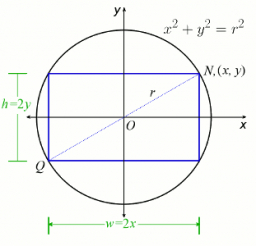# The rectangle 4

The rectangle above has a length of 7x and a width of 3. The perimeter of the rectangle is represented by the algebraic expression 14x + 6. Find the perimeter of the rectangle when x = 2.

p =  34

### Step-by-step explanation:Did you find an error or inaccuracy? Feel free to write us. Thank you!

#### Grade of the word problem:

We encourage you to watch this tutorial video on this math problem: